# Defining and Simulating Populations

##### 2021-08-11
using Random
using Pumas
using PumasUtilities


# Introduction

In this tutorial, we will cover the fundamentals of generating populations to simulate with Pumas. We will demonstrate how to specify dosage regimens and covariates, and then how to piece these together to form a population to simulate.

## The model

Below is a Pumas model that specifies a 1-compartment oral absorption system with between-subject variability on all the parameters. Details of the model specification are provided in the documentation.

model = @model begin
@param begin
θ ∈ VectorDomain(4)
Ω ∈ PSDDomain(3)
σ²_prop ∈ RealDomain(init=0.1)
end

@random begin
η ~ MvNormal(Ω)
end

@covariates Wt

@pre begin
CL = θ*(Wt/70)^0.75*exp(η)
V = θ*(Wt/70)^0.75*exp(η)
Ka = θ*exp(η)
end

@dynamics begin
Depot'   = -Ka*Depot
Central' =  Ka*Depot - Central*CL/V
end

@derived begin
conc = @. Central/V
dv ~ @.Normal(conc,sqrt(conc^2*σ²_prop))
end

end

PumasModel
Parameters: θ, Ω, σ²_prop
Random effects: η
Covariates: Wt
Dynamical variables: Depot, Central
Derived: conc, dv
Observed: conc, dv


## Setting up parameters

Next we provide the initial estimates of the parameters to simulate from. The fixed effects are provided in the θ vector (CL, V, Ka) and the between-subject variability parameteres are provided in the Ω vector as variances. So, 0.04 variance on Ω11 suggests a 20% coefficient of variation. Similarly, σ²prop has a 20% proportional residual error.

fixeffs = (
θ = [0.4,20,1.1,2],
Ω = diagm(0 => [0.04,0.04,0.04]),
σ²_prop = 0.04
)

(θ = [0.4, 20.0, 1.1, 2.0], Ω = [0.04 0.0 0.0; 0.0 0.04 0.0; 0.0 0.0 0.04],
σ²_prop = 0.04)


## Single dose example

DosageRegimen() is the function that lets you construct a dosing regimen. The first argument of the DosageRegimen is amt and is not a named argument. All subsequent arguments need to be named. Lets try a simple example where you provide a 100 mg dose at time = 0.

ev = DosageRegimen(100, time = 0)
first(ev.data)


DataFrameRow (10 columns)

Float64Int64Float64Int8Float64Int64Float64Float64Int8Route…
10.01100.010.000.00.00NullRoute

As you can see above, we provided a single 100 mg dose. DosageRegimen provides some defaults when it creates the dataset, time = 0, evid = 1, cmt = 1, rate = 0, ii = 0 & addl = 0.

Note that ev is of type DosageRegimen. Specified like above, DosageRegimen is one of the four fundamental building block of a Subject (more on Subject below).

### Building Subjects

Let's create a single subject

s1 = Subject(id = 1,
events = ev,
covariates = (Wt = 70,),
observations = (dv = nothing,))

Subject
ID: 1
Events: 1
Observations: dv: (nothing)
Covariates: Wt


Note that the s1 Subject created above is composed of:

• id: an unique identifier

• observations: observations, represented by Pumas.Observation

• covariates: covariates, represented by Pumas.ConstantCovar

• events: events, represented by Pumas.Event

DataFrame(s1.events)


1 rows × 11 columns (omitted printing of 1 columns)

amttimeevidcmtratedurationssiibase_timerate_dir
Float64Float64Int8Int64Float64Float64Int8Float64Float64Int8
1100.00.0110.0Inf00.00.01

The events are presented by basic information such as the dose of drug, the time of dose administration, the compartment number for administration and whether the dose is an instantaneous input or an infusion.

Below is how the covariates are represented, as a NamedTuple.

s1.covariates

Pumas.ConstantCovar{NamedTuple{(:Wt,), Tuple{Int64}}}((Wt = 70,))


(Note: defining distributions for covariates will be discussed in detail later.)

Using this one subject, s1, let us simulate a simple concentration time profile using the model above:

Random.seed!(1234)
sims = simobs(model,
s1,
fixeffs,
obstimes = 0:0.1:120)


and plot the result

sim_plot(model,
[sims], observations = [:conc],
figure = (fontsize = 18, ),
axis = (xlabel = "Time (hr)",
ylabel = "Predicted Concentration (ng/mL)"))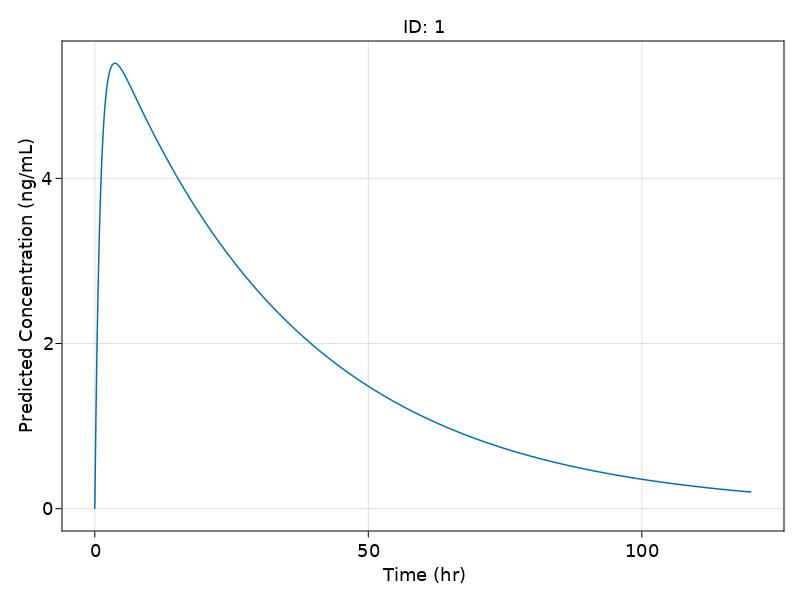### Building Populations

Now, lets create one more subject, s2.

s2 = Subject(id = 2,
events = ev,
covariates = (Wt = 70,),
observations = (dv = nothing,))

Subject
ID: 2
Events: 1
Observations: dv: (nothing)
Covariates: Wt


If we want to simulate both s1 and s2 together, we need to bring these subjects together to form a Population. A Population is essentially a collection of subjects.

twosubjs =  [s1,s2]

Population
Subjects: 2
Covariates: Wt
Observations: dv


Let's see the details of the first and the second subject

twosubjs

Subject
ID: 1
Events: 1
Observations: dv: (nothing)
Covariates: Wt

twosubjs

Subject
ID: 2
Events: 1
Observations: dv: (nothing)
Covariates: Wt


Now, we can simulate this Population of 2 subjects as below

Random.seed!(1234)
sims = simobs(model,
twosubjs,
fixeffs,
obstimes = 0:0.1:120)


and plot the result

sim_plot(model,
sims, observations = [:conc],
figure = (fontsize = 18, ),
axis = (xlabel = "Time (hr)",
ylabel = "Predicted Concentration (ng/mL)"))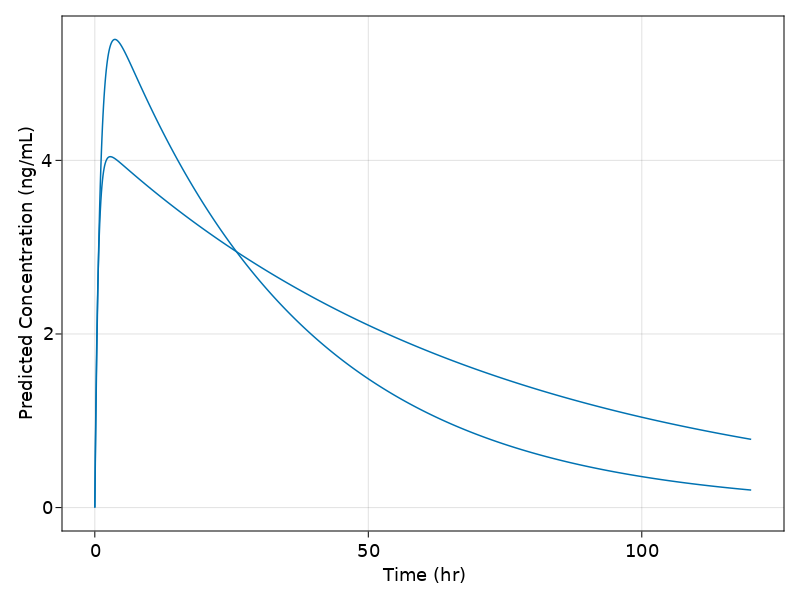Similarly, we can build a population of any number of subjects. But before we do that, let's dive into covariate generation.

### Covariates

As was discussed earlier, a Subject can also be provided details regarding covariates. In the model above, a continuous covariate body weight Wt impacts both CL and V. Let us now specify covariates to a population of 10 subjects.

Random.seed!(1234)
choose_covariates() = (Wt = rand(55:80),)

choose_covariates (generic function with 1 method)


choose_covariates will randomly choose a Wt between 55-80 kgs

We can make a list with covariates for ten subjects through a list comprehension

cvs = [ choose_covariates() for i in 1:10 ]
DataFrame(cvs)


10 rows × 1 columns

Wt
Int64
167
270
379
470
558
666
778
858
958
1073

Now, we add these covariates to the population as below. The map(f,xs) will return the result of f on each element of xs. Let's map a function that build's a subject with the randomly chosen covariates in order to build a population:

pop_with_covariates = map(i -> Subject(id = i,
events = ev,
covariates = choose_covariates(),
observations = (dv = nothing,)), 1:10)

Population
Subjects: 10
Covariates: Wt
Observations: dv


Simulate into the population

Random.seed!(1234)
sims = simobs(model,
pop_with_covariates,
fixeffs,
obstimes = 0:0.1:120)


and visualize the output

sim_plot(model,
sims, observations = [:conc],
figure = (fontsize = 18, ),
axis = (xlabel = "Time (hr)",
ylabel = "Predicted Concentration (ng/mL)"))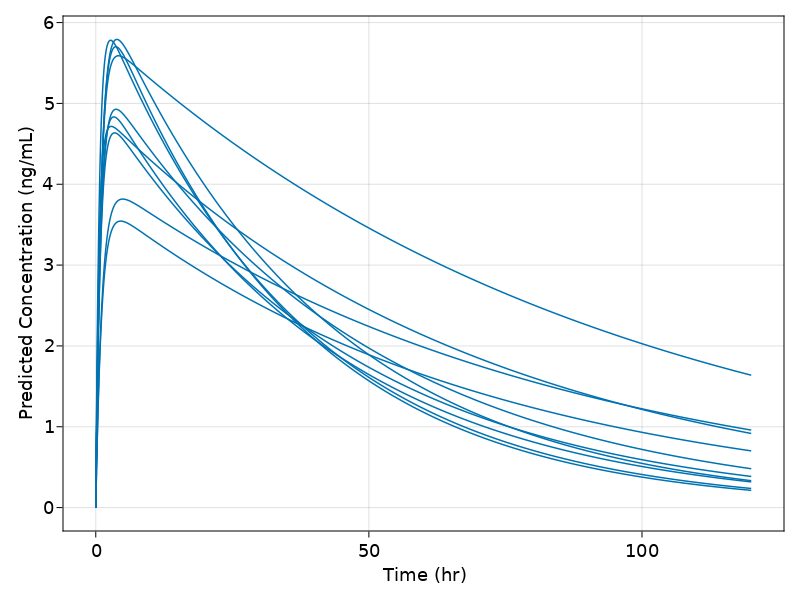## Multiple dose example

DosageRegimen provides additional controls over the specification of the regimen. For example, ii defines the "interdose interval", or the time difference between two doses, while addl defines how many additional times to repeat a dose. Thus, let's define a dose of 100 that's repeated 7 times at 24 hour intervals:

md =  DosageRegimen(100, ii = 24, addl = 6)


1 rows × 10 columns

Float64Int64Float64Int8Float64Int64Float64Float64Int8Route…
10.01100.0124.060.00.00NullRoute

Let's create a new subject, s3 with this dosage regimen:

s3 = Subject(id = 3,
events = md,
covariates = (Wt = 70,),
observations = (dv = nothing,))

Subject
ID: 3
Events: 7
Observations: dv: (nothing)
Covariates: Wt


and see the results:

Random.seed!(1234)
sims = simobs(model,
s3, fixeffs,
obstimes = 0:0.1:240)

sim_plot(model,
[sims], observations = [:conc],
figure = (fontsize = 18, ),
axis = (xlabel = "Time (hr)",
ylabel = "Predicted Concentration (ng/mL)"))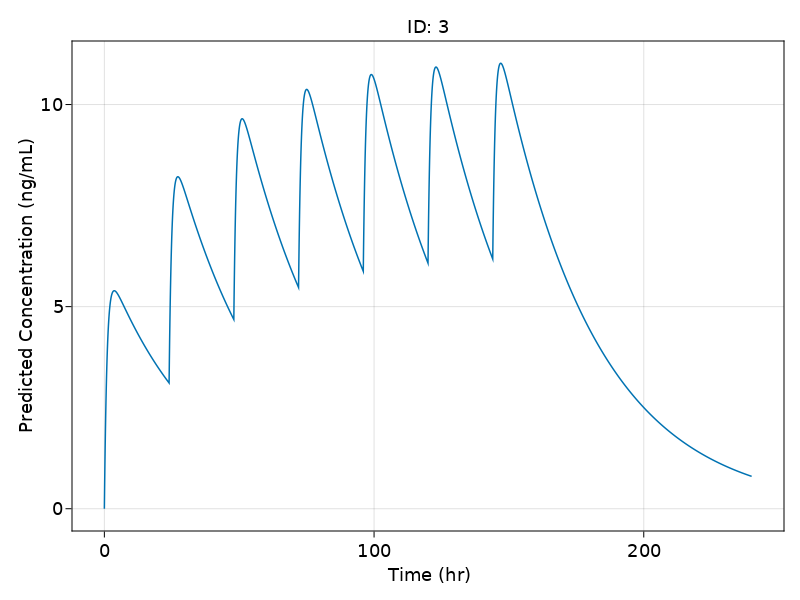## Combining dosage regimens

We can also combine dosage regimens to build a more complex regimen. Recall from the introduction that using arrays will build the element-wise combinations. Thus let's build a dose of 500 into compartment 1 at time 0, and 7 doses into compartment 1 of 100 spaced by 24 hours:

ldmd = DosageRegimen([500,100],
time = [0,24],
cmt = 1,
ii = [0,24])


2 rows × 10 columns

Float64Int64Float64Int8Float64Int64Float64Float64Int8Route…
10.01500.010.000.00.00NullRoute
224.01100.0124.060.00.00NullRoute

Let's see if this result matches our intuition:

s4 = Subject(id = 4,
events = ldmd,
covariates = (Wt = 70,),
observations = (dv = nothing,))
Random.seed!(1234)
sims = simobs(model,
s4,
fixeffs,
obstimes = 0:0.1:120)

sim_plot(model,
[sims], observations = [:conc],
figure = (fontsize = 18, ),
axis = (xlabel = "Time (hr)",
ylabel = "Predicted Concentration (ng/mL)"))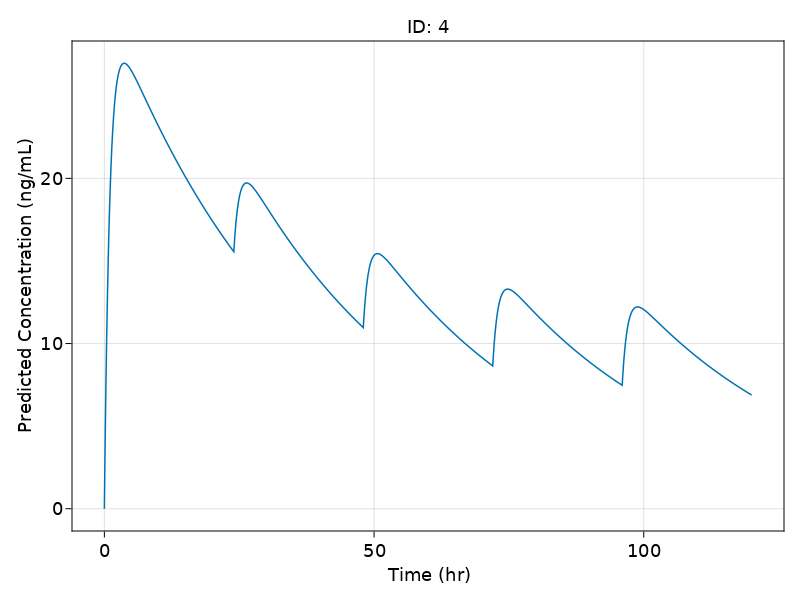Another way to build complex dosage regiments is to combine previously constructed regimens into a single regimen. For example:

e1 = DosageRegimen(500,cmt = 1, time = 0, addl = 0, ii = 0)
e2 = DosageRegimen(100,cmt = 1, time = 24, addl = 6, ii = 24)
evs = DosageRegimen(e1,e2)
s5 = Subject(id = 5,
events = evs,
covariates = (Wt = 70,),
observations = (dv = nothing,))
Random.seed!(1234)
sims = simobs(model,
s5,
fixeffs,
obstimes = 0:0.1:120)

sim_plot(model,
[sims], observations = [:conc],
figure = (fontsize = 18, ),
axis = (xlabel = "Time (hr)",
ylabel = "Predicted Concentration (ng/mL)"))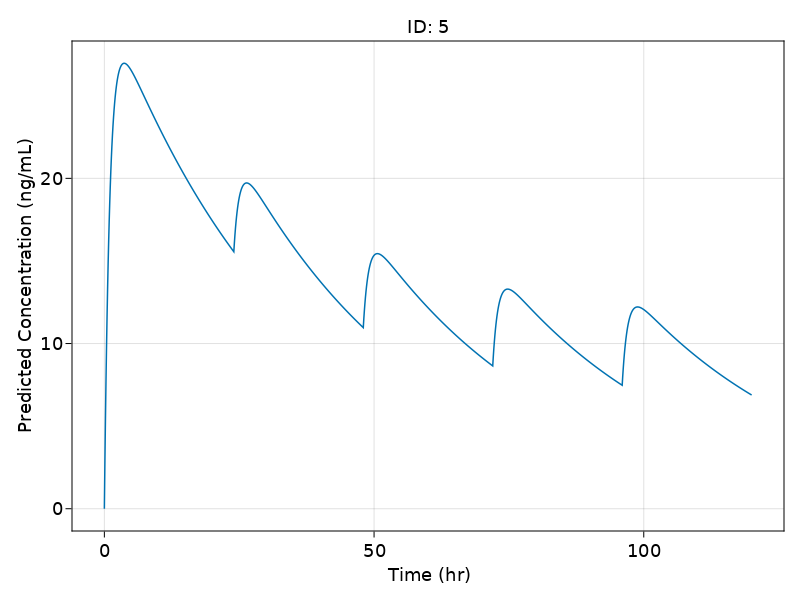is the same regimen as before.

Putting these ideas together, we can define a population where individuals with different covariates undergo different regimens, and simulate them all together:

e1 = DosageRegimen(100, ii = 24, addl = 6)
e2 = DosageRegimen(50,  ii = 12, addl = 13)
e3 = DosageRegimen(200, ii = 24, addl = 2)


1 rows × 10 columns

Float64Int64Float64Int8Float64Int64Float64Float64Int8Route…
10.01200.0124.020.00.00NullRoute
pop1 = map(i -> Subject(id = i, events = e1, covariates = choose_covariates(),
observations = (dv = nothing,)), 1:5)
pop2 = map(i -> Subject(id = i, events = e2, covariates = choose_covariates(),
observations = (dv = nothing,)), 6:8)
pop3 = map(i -> Subject(id = i, events = e3, covariates = choose_covariates(),
observations = (dv = nothing,)), 9:10)
pop  = vcat(pop1, pop2, pop3)

Population
Subjects: 10
Covariates: Wt
Observations: dv

Random.seed!(1234)
sims = simobs(model,
pop,
fixeffs,
obstimes = 0:0.1:120)

sim_plot(model,
sims, observations = [:conc],
figure = (fontsize = 18, ),
axis = (xlabel = "Time (hr)",
ylabel = "Predicted Concentration (ng/mL)"))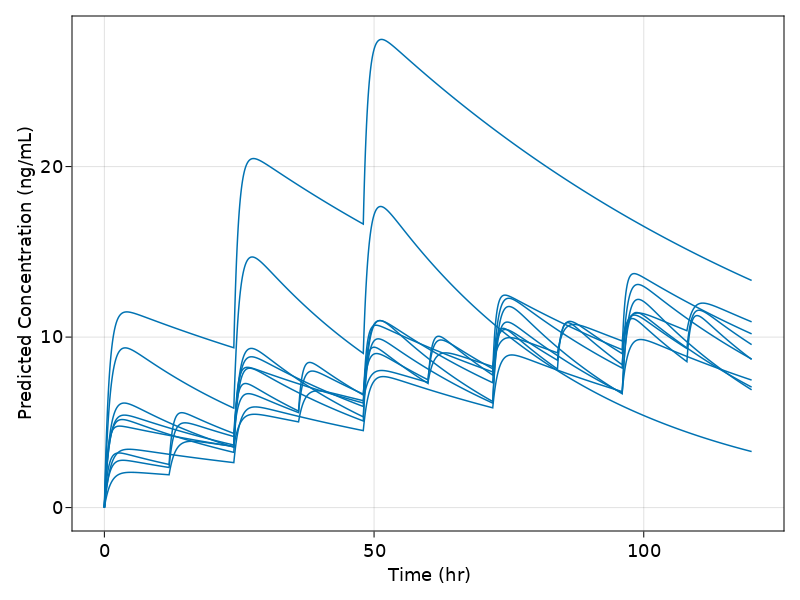## Defining Infusions

An infusion is a dosage which is defined as having a non-zero positive rate at which the drug enters the system. Let's define a single infusion dose of total amount 100 with a rate of 3 unit amount per time into the second compartment:

inf = DosageRegimen(100, rate = 3, cmt = 2)


1 rows × 10 columns

Float64Int64Float64Int8Float64Int64Float64Float64Int8Route…
10.02100.010.003.033.33330NullRoute

Now let's simulate a subject undergoing this treatment strategy:

s6 = Subject(id = 5,
events = inf,
covariates = (Wt = 70,),
observations = (dv = nothing,))
Random.seed!(1234)
sims = simobs(model,
s6,
fixeffs,
obstimes = 0:0.1:120)

sim_plot(model,
[sims], observations = [:conc],
figure = (fontsize = 18, ),
axis = (xlabel = "Time (hr)",
ylabel = "Predicted Concentration (ng/mL)"))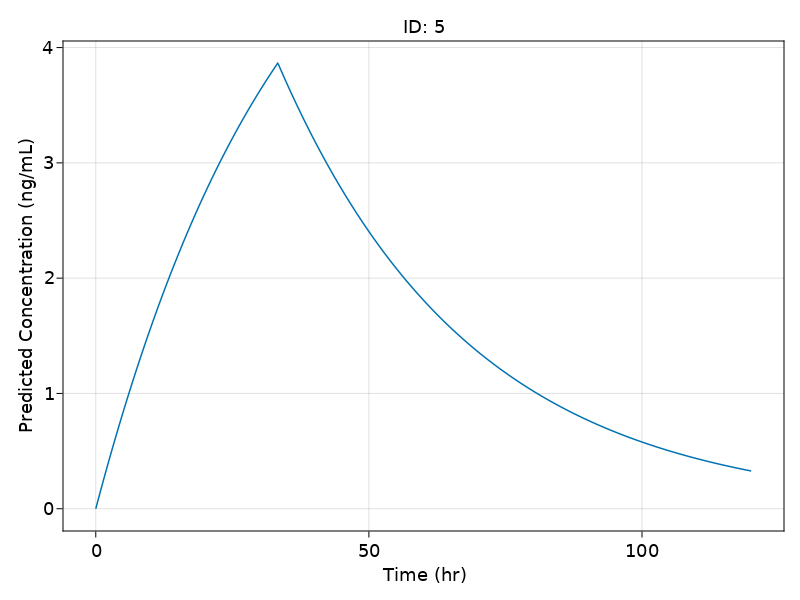## Final Note on Julia Programming

Note that all of these functions are standard Julia functions, and thus standard Julia programming constructions can be utilized to simplify the construction of large populations. We already demonstrated the use of map and a comprehension, but we can also make use of constructs like for loops.

## Conclusion

This tutorial shows the tools for generating populations of infinite complexity, defining covariates and dosage regimens on the fly and simulating the results of the model.